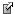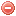# Software

We developed a tool to calculate some fire weather indices from meteorological parameters, consisting of a library, a command line application and a graphical interface application.
We used the Scalalanguage, which is targetting the Java virtual machine (jvm).

This tool reads meteorological data from a *.csv file and calculates the fire weather indices according to the inputs, storing the results in another *.csv file and the details of the calculation in a text file (log). For each index multiple possible input parameters have been specified, according to a ranking from the optimal to the less suitable choice. For example for the canadian FWI if the parameters at noon are not available, the program will try to compute it with other meaningful daily values. Variable choice is indicated in the logs.

# Input file

The program can read *.csv files (comma separated values), with headers with a specific name (columns with other names will not be considered). The case (upper/lower) is not relevant. For a list of the abbreviations used see the Variable section. The values are checked for missing values and range validity. The values of the input variables wich are out of range are replaced with a missing value (not in the original file, only to perform the calculations).

# Settings

You can change the settings according to your local situation. In the missing variables tab you can specify some parameters which can be used for the calculation as a replacement. The parameter can also be imported form a file (see Preferences).

# Preferences

You can specify if the parameters and missing variables should be read from the ui (user interface), a parameter file (*_PAR.txt) or both (file parameters have the precedence over ui parameters). The file option allows you to use different parameters for different stations in the multi file calculation. The parameters in the *_PAR.txt file should be specified as the following example:
Altitude 500
Latitude 46.0
FireSeasonStart 15.01
FireSeasonEnd 15.10
Climate 3

# Output files

The program generates two files, one containing the results (xxx_RESULTS.csv) and one reporting the log (xxx_LOG.txt), which are stored in the same directory as the input file (xxx.csv). For each calculated variable, a log is given with the calclulation details:
start: initial value
(required variables)->(used variables)
[parameters used]

# Parameters

Here is a list of the parameters and missing variables that can be specified in a *_PAR.txt file (see paragraph on preferences).

 Altitude Altitude m Latitude Latitude ° Krs Krs Albedo Albedo RainyDayThreshold Threshold for rainy day mm KBDIstart KBDIstart FFMCstart MFFMCstart DMCstart DMCstart DCstart DCstart FireSeasonstart Start of the fire season day-month FireSeasonEnd End of the fire season day-month M68VegCorrStep3Start vegCorrStep3Start M68VegCorrStep3End vegCorrStep3End -- XsnowcoverStart XsnowcoverStart day-month XsnowcoverEnd XsnowcoverEnd day-month XbirchLeaves XbirchLeaves day-month XrobiniaBlossom XrobiniaBlossom day-month MeanAnnualRain Mean annual rain mm/year Climate Climate class (Deeming et al. 1977) 1-4 I Heat index

# Variables

Here is a list of the variables used with the abbreviations, units and variables required for the computation.

## Inputs:

 DateYYYYMMDD e.g.  20081231 T Temperature average °C T12 Temperature at 12:00 °C T13 Temperature at 13:00 °C T15 Temperature at 15:00 °C Tmin Minimal temperature °C Tmax Maximal temperature °C P Rainfall sum at 06:00 mm/day P12 Rainfall sum at 12:00 mm/day P13 Rainfall sum at 13:00 mm/day P15 Rainfall sum at 15:00 mm/day U Windspeed average m/s U12 Windspeed at 12:00 m/s U13 Windspeed at 13:00 m/s U15 Windspeed at 15:00 m/s H Relative humidity % H12 Relative humidity at 12:00 % H13 Relative humidity at 13:00 % H15 Relative humidity at 15:00 % Hmin Minimum Relative humidity % Hmax Maximum Relative humidity % Cc Cloud cover fraction ratio

## Optional inputs:

 SnowCover Snow cover 0/1 (T, XsnowcoverStart, XsnowcoverEnd) BirchLeaves Phenological phase first birch leaves 0/1 (T, XbirchLeaves) RobiniaBlossom Phenological phase robinia blossom 0/1 (T, XrobiniaBlossom) PC Phenological coefficient 100/200 (r) PDur Duration of rainfall in a day h (P, Climate)

## Calculables: (from inputs or settings)

 Tdew Dewpoint temperature °C (T, H) Tdew12 Dewpoint temperature at 12:00 °C (T12, H12) Tdew15 Dewpoint temperature at 15:00 °C (T15, H15) PDur Duration of rainfall in a day h (P, Climate) SnowCover Snow cover 0/1 (T, XsnowcoverStart, XsnowcoverEnd) RobiniaBlossom Phenological phase robinia blossom 0/1 (T, XrobiniaBlossom) BirchLeaves Phenological phase first birch leaves 0/1 (T, XbirchLeaves) PC Phenological coefficient 100/200 (r) N Daylight hours FAO day (T, Latitude) DaysSinceRain Days since last rainfall day (P, RainyDayThreshold) AgeRainEvent_2_20 Age of last significant rainfall event (days > 2mm in past 20 days)(day with max rain in event) days (P) WeekRain One week rainfall mm/week (P) RainSum Sum of consecutive rainfall mm (P) lastRainSum Sum of last consecutive rainfall mm (P) lastRainSum_2_20 Sum of last significant rainfall event (days > 2mm in past 20 days) mm (P) VPD Vapour pressure deficit value kPa (Tmax, Tmin, H) VPD13 Vapour pressure deficit value at 13:00 kPa (T13, H13) Angstroem Angstroem index value — (T, H) Nesterov Nesterov index value — (T15, Tdew15, P15) Munger Munger index value — (P) EMC Equilibrium moisture content % (T, H) EMCfa Equilibrium moisture content at fuel-atmosphere interface % (T, H, Cc) EMC24 Weighted 24-hour average EMC % (Tmin, Tmax, Hmin, Hmax, N) FFWI FFWI index value — (EMC, U) FFWImod modified FFWI index value — (KBDI, FFWI) KBDI KBDI index value inches/100 (Tmax, P, MeanAnnualRain, KBDIstart, RainSum, WeekRain, RainyDayThreshold) KBDISI KBDISI index value mm (Tmax, P, MeanAnnualRain, KBDIstart, RainSum, WeekRain, RainyDayThreshold) Sharples Sharples index value — (T, H, U) FMI Sharples fuel moisture index — (T, H) Baumgartner Baumgartner index — (P, PETpen) BaumgartnerDanger Baumgartner Danger classes — (Baumgartner) NetRad Net Radiation — (H, Tmax, Tmin, Latitude, Altitude, Krs, Albedo) PETpen Potential evapotranspiration value Penmann formula mm/day (NetRad, T, U, VPD, Altitude) PETthorn Potential evapotranspiration value Thorntwaite formula mm/day (T, I, N) PETthorn_camargo Potential evapotranspiration value Thorntwaite formula, with Tef of Camargo et al (1999) mm/day (Tmin, Tmax, I, N) PETthorn_pereira Potential evapotranspiration value Thorntwaite formula, with Tef of Pereira and Pruitt (2004) mm/day (Tmin, Tmax, I, N) r soil water reserve (Orieux Index) mm (P, PETthorn, WeekRain, KBDIstart) OrieuxDanger Orieux Danger classes (r, U) rs surface soil water reserve (Carrega 1988) mm (P, PETthorn, RainSum) I87 I87 index (Carrega 1988) — (T, H, U, P, r, rs, PC) RN Numerical risk index — (T, Tdew, U, Cc, r) M68 M68 index value — (pM68, P, BirchLeaves, RobiniaBlossom, M68VegCorrStep3Start, M68VegCorrStep3End) pM68 pM68 index value — (T13, VPD13, P13, SnowCover, FireSeasonstart, FireSeasonEnd) M68dwd M68 index value (DWD modification) — (pM68dwd, P, BirchLeaves, RobiniaBlossom, M68VegCorrStep3Start, M68VegCorrStep3End) pM68dwd pM68 index value (DWD modification) — (T13, H13, P13, SnowCover, FireSeasonstart, FireSeasonEnd) FFDI MacArthur5 index value — (DFgriffithAdj, T15, H15, U15) DFnoble Drought factor (Noble 1980) — (lastRainSum, DaysSinceRain, KBDISI) DFgriffith Drought factor (Griffith 1999) — (lastRainSum_2_20, AgeRainEvent_2_20, KBDISI) DFgriffithAdj Drought factor (Finkele et al.2006) — (lastRainSum_2_20, AgeRainEvent_2_20, KBDISI) FWI Fire weather index — (BUI, ISI) ISI Initial spread index — (FFMC, U12) BUI Buildup index — (DMC, DC) FFMC Fine fuel moisture code — (T12, H12, U12, P12, SnowCover, FFMCstart) DMC Duff moisture code — (T12, H12, P12, SnowCover, DMCstart) DC Drought code — (T12, P12, SnowCover, DCstart) MC1 1-hour timelag fuel moisture % (EMCfa) MC10 10-hour timelag fuel moisture % (EMCfa) MC100 100-hour timelag fuel moisture % (EMC24, PDur) MC1000 1000-hour timelag fuel moisture % (EMC24, PDur) Ifa Ifa index (Portuguese Index) — (T12, Tdew12, P, U12, FireSeasonstart, FireSeasonEnd)

### Bibliography SearchNo results!

No results were found. Are you sure you searched for a tag?

### Symbols

 Variable Description Unit $$T$$ air temperature °C $$T_{dew}$$ dew point temperature °C $$H$$ air humidity % $$P$$ rainfall mm $$U$$ windspeed m/s $$w$$ days since last rain (or rain above threshold) d $$rr$$ days with consecutive rain d $$\Delta t$$ time increment d $$\Delta{e}$$ vapor pressure deficit kPa $$e_s$$ saturation vapor pressure kPa $$e_a$$ actual vapor pressure kPa $$p_{atm}$$ atmospheric pressure kPa $$PET$$ potential evapotranspiration mm/d $$r$$ soil water reserve mm $$r_s$$ surface water reserve mm $$EMC$$ equilibrium moisture content % $$DF$$ drought factor - $$N$$ daylight hours hr $$D$$ weighted 24-hr average moisture condition hr $$\omega$$ sunset hour angle rad $$\delta$$ solar declination rad $$\varphi$$ latitude rad $$Cc$$ cloud cover Okta $$J$$ day of the year (1..365/366) - $$I$$ heat index - $$R_n$$ net radiation MJ⋅m-2⋅d-1 $$R_a$$ daily extraterrestrial radiation MJ⋅m-2⋅d-1 $$R_s$$ solar radiation MJ⋅m-2⋅d-1 $$R_{so}$$ clear-sky solar radiation MJ⋅m-2⋅d-1 $$R_{ns}$$ net shortwave radiation MJ⋅m-2⋅d-1 $$R_{nl}$$ net longwave radiation MJ⋅m-2⋅d-1 $$\lambda$$ latent heat of vaporization MJ/kg $$z$$ elevation m a.s.l. $$d_r$$ inverse relative distance Earth-Sun - $$\alpha$$ albedo or canopy reflection coefficient - $$\Delta$$ slope of the saturation vapor pressure curve kPa/°C $$Cc$$ cloud cover eights $$ROS$$ rate of spread m/h $$RSF$$ rate of spread factor - $$WF$$ wind factor - $$WRF$$ water reserve factor - $$FH$$ false relative humidity - $$FAF$$ fuel availability factor - $$PC$$ phenological coefficient -

 Suffix Description $$-$$ mean / daily value $$_{max}$$ maximum value $$_{min}$$ minimum value $$_{12}$$ value at 12:00 $$_{13}$$ value at 13:00 $$_{15}$$ value at 15:00 $$_{m}$$ montly value $$_{y}$$ yearly value $$_{f/a}$$ value at fuel-atmosphere interface $$_{dur}$$ duration $$_{soil}$$ value at soil level

 Constant Description $$e$$ Euler's number $$\gamma$$ psychrometric constant $$G_{SC}$$ solar constant $$\sigma$$ Stefan-Bolzmann constant